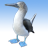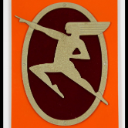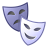# Label Expression to not label if...

630
5
09-29-2010 08:38 AMOccasional Contributor II
I am wondering how to get my label expression to not label a field if certain other fields are found.
Below is my expression.

```Function FindLabel ( [LTSA_LOT] , [LTSA_BLOCK] , [LTSA_PA4] , [LTSA_PLAN] , [LAND_AC6] )
if ( Len ([LTSA_LOT]) <> 0) then
str1 = [LTSA_LOT] & " - "
end if
if ( Len ( [LTSA_BLOCK] )  <> 0) then
str2 =  [LTSA_BLOCK] & " - "
end if
if ( Len ( [LTSA_PA4] )  <> 0) then
str3 =  [LTSA_PA4] & " - "
end if
if ( Len( [LTSA_PLAN] ) <> 0) then
str4 =  [LTSA_PLAN]
end if
if ( Len( [LAND_AC6] ) <> 0) then
str5 =  [LAND_AC6]
end if
FindLabel = str1 & str2 & str3 & str4 & VBNewLine & str5
End Function```

I would like the label to not label Str5, if labels are found for str1-4.

Appreciate your time to help me with this.
Tags (2)
5 RepliesbyMVP Honored Contributor
Here are two ways of looking at it. If you want to add the attribute for LAND_AC6 only when none of the other fields have any attributes, then use this.

```Function FindLabel ( [LTSA_LOT] , [LTSA_BLOCK] , [LTSA_PA4] , [LTSA_PLAN] , [LAND_AC6] )

if ( Len ([LTSA_LOT]) <> 0) then
str = [LTSA_LOT] & " - "
end if
if ( Len ( [LTSA_BLOCK] )  <> 0) then
str = str + [LTSA_BLOCK] & " - "
end if
if ( Len ( [LTSA_PA4] )  <> 0) then
str = str +  [LTSA_PA4] & " - "
end if
if ( Len( [LTSA_PLAN] ) <> 0) then
str = str +   [LTSA_PLAN]
end if
if ( Len( [LAND_AC6] ) <> 0) then
str1 =  [LAND_AC6]
end if

if (Len(str) > 0) then
FindLabel = str
else
FindLabel = str1
end if

End Function
```

If you want to add the attribute for LAND_AC6 if one or more of the other fields don't have any attributes, then this should work
```Function FindLabel ( [LTSA_LOT] , [LTSA_BLOCK] , [LTSA_PA4] , [LTSA_PLAN] , [LAND_AC6] )

dim found
found = True

if ( Len ([LTSA_LOT]) <> 0) then
str = [LTSA_LOT] & " - "
else
found = False
end if
if ( Len ( [LTSA_BLOCK] )  <> 0) then
str = str + [LTSA_BLOCK] & " - "
else
found = False
end if
if ( Len ( [LTSA_PA4] )  <> 0) then
str = str +  [LTSA_PA4] & " - "
else
found = False
end if
if ( Len( [LTSA_PLAN] ) <> 0) then
str = str +   [LTSA_PLAN]
else
found = False
end if
if ( Len( [LAND_AC6] ) <> 0) then
str1 =  [LAND_AC6]
end if

if found then
FindLabel = str
else
FindLabel = str & vbNewLine & str1
end if
End Function
```Occasional Contributor II
Perfect, thanks Ken,

never thought of the Found=False parameter or setting desired labels to one str!New Contributor
I'm having some trouble. I have two fields that I want to label because on some rows, the data is different. What would the expression be if I wanted to label both fields but tell it not to label second field if values are the same? Thanks in advance!Occasional Contributor II
...What would the expression be if I wanted to label both fields but tell it not to label second field if values are the same?...

Check out this similar thread from the Cartotalk forum to compare two fields for matching records with some options for a label expression.

```Function FindLabel ( [F1], [F2]  )
intComp = StrComp( [F1], [F2], vbTextCompare )
if intComp = -1 Then
FindLabel = [F1]
Else
FindLabel = [F1] & " - " & [F2]
End if
End Function```

Results:
[ATTACH]7782[/ATTACH]

In the expression the logic is: if they match, label only [F1], but if they do not match, label [F1] and [F2]Occasional Contributor II
What would the expression be if I wanted to label both fields but tell it not to label second field if values are the same?

I went over a way how to compare two fields for matching values in a label expression over on the Cartotalk.

There is another method mentioned in there, but using the StrComp function you would need to make a small change to the expression:

```Function FindLabel ( [F1], [F2]  )
intComp = StrComp( [F1], [F2], vbTextCompare )
if intComp = -1 Then
FindLabel = [F1] & " - " & [F2]
Else
FindLabel = [F1]
End if
End Function```

Results:
[ATTACH]7784[/ATTACH]